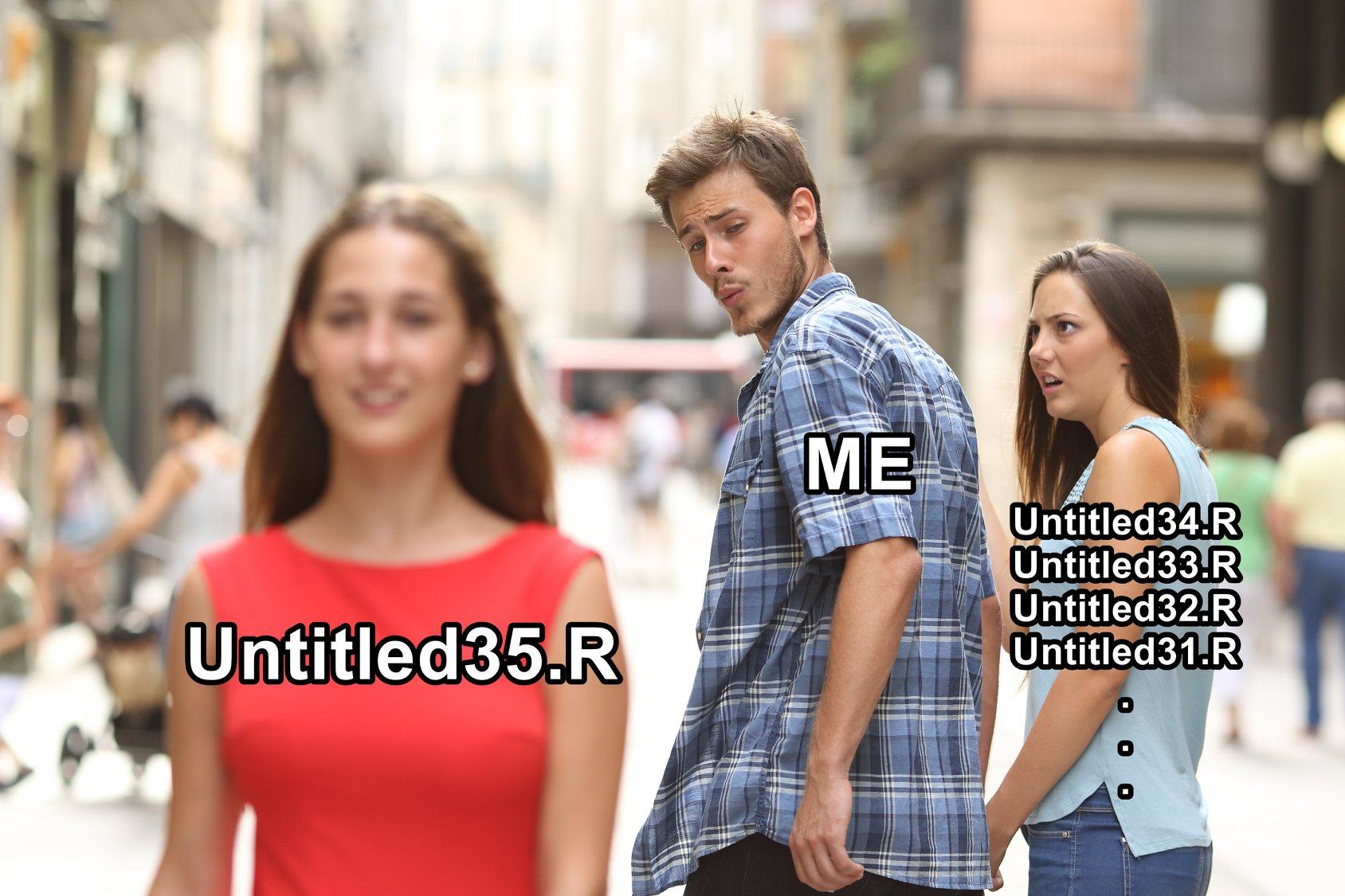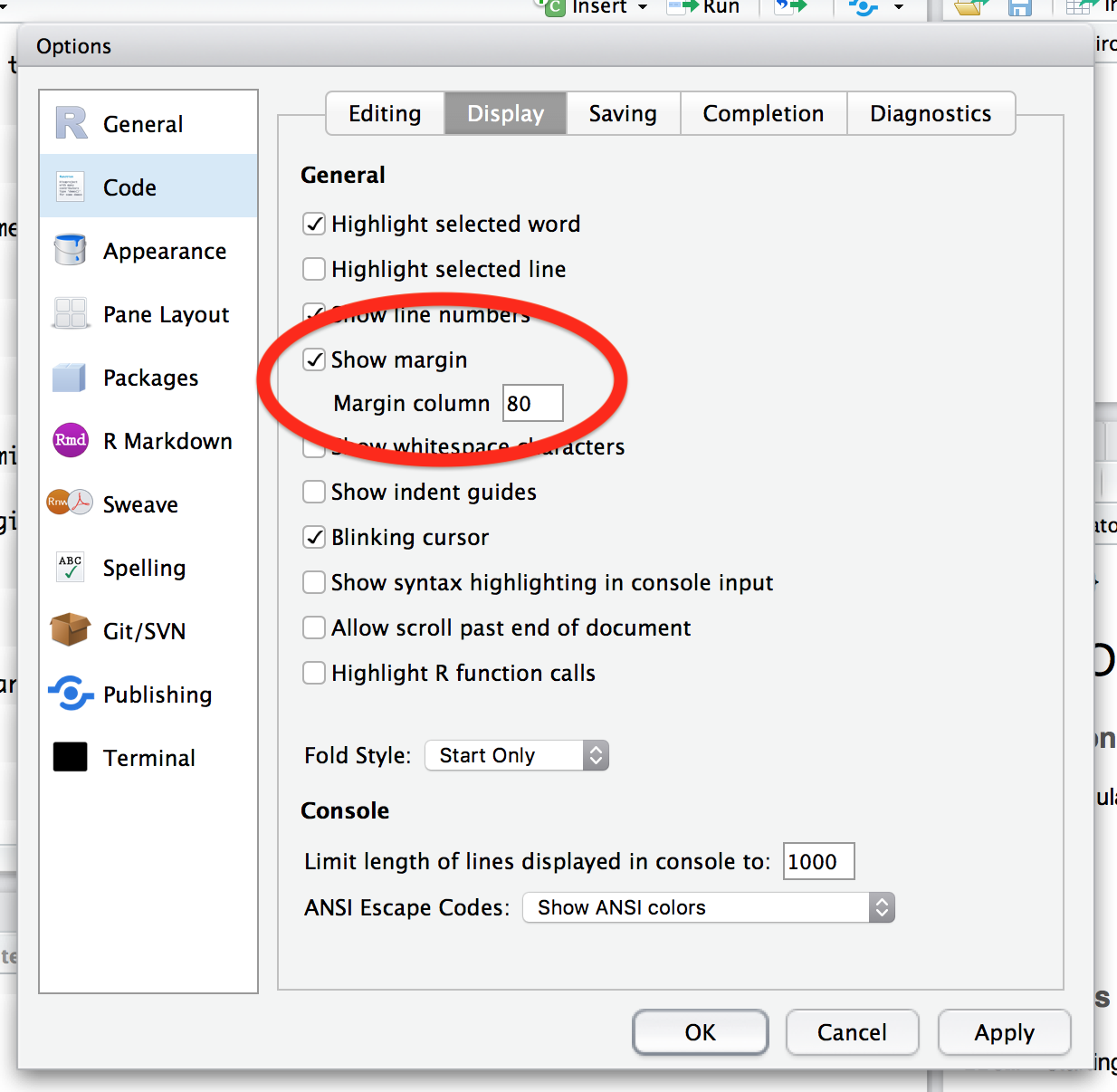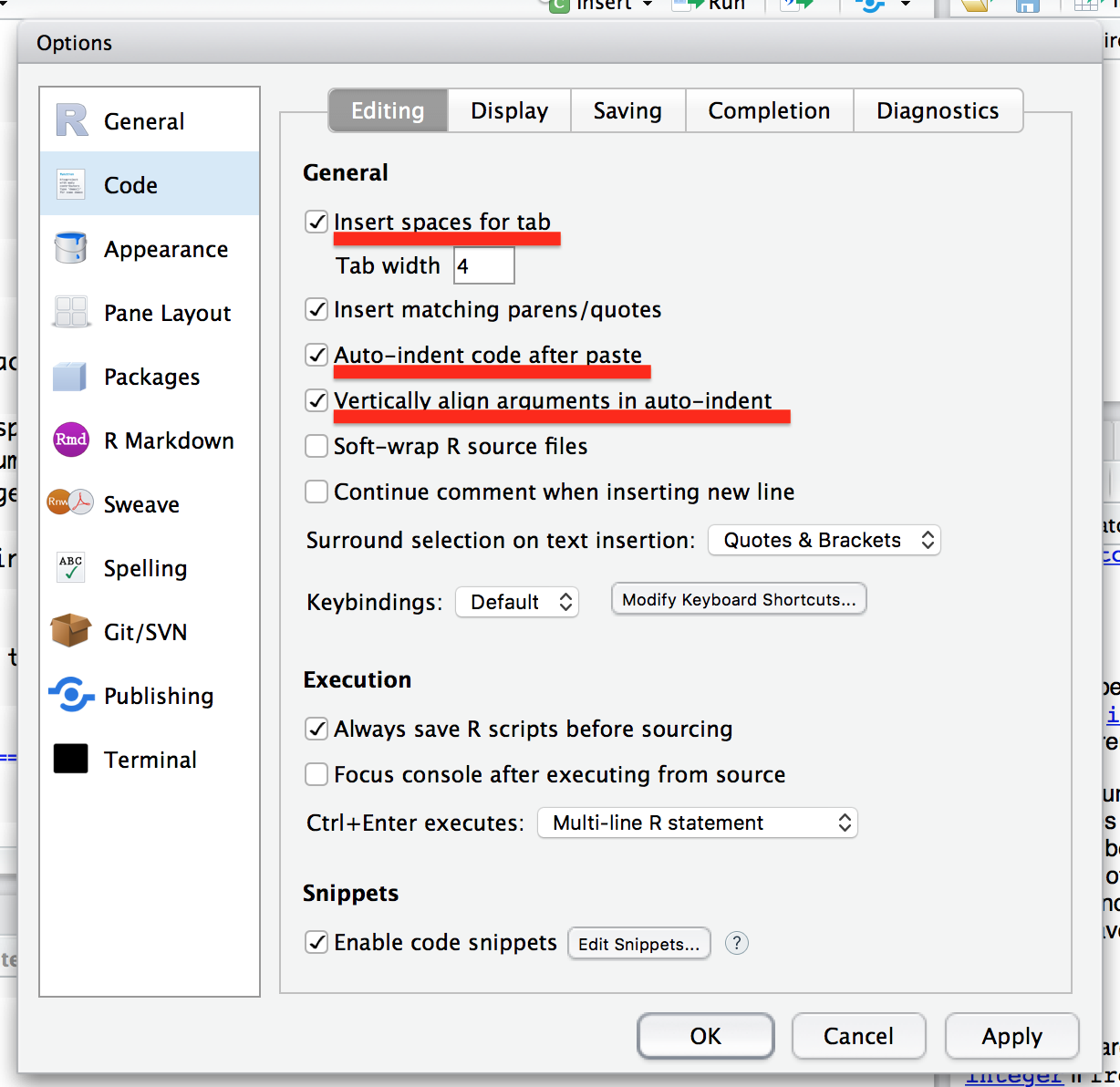# R Coding Style Guide

Good coding style is like using correct punctuation. You can manage without it, but it sure makes things easier to read. Hadley Wickham

### Introduction

A language is a tool that allows human beings to interact and communicate with each other. The clearer we express ourselves, the better an idea is transferred from our mind to the other. The same applies to programming languages: concise, clear and consistent codes are easier to read and/or edit. It is especially important, if you have collaborators, who depend on your code. However, even if you don’t, keep in mind that at some point in time, you might come back to your code, for example, to fix an error. And if you did not follow consistently your coding style, reviewing your code can take much longer, than you expected. In this context, taking care of your audience means to make your code as readable as possible.

There is no such thing as a “correct” coding style, as there is no such thing as the best color. At the end of the day, the coding style is a set of developers’ preferences. If you are coding alone, sticking to your coding style and being consistent is more than enough. The story is a bit different if you are working in a team: it is crucial to agree on a convention beforehand and make sure that everyone follows it.Even though there is no an official style guide, R is mature and steady enough to have an “unofficial” convention. In this tutorial, you will learn these “unofficial” rules, their deviations, and most common styles.

### Naming

#### Naming files

The convention actually depends on whether you develop a file for a package, or as a part of data analysis process. There are, however, common rules:

• File names should use .R extension.

# Good

read
• File names should be meaningful.

# Good
model.R

Untitled1.R
• File names should not contain / and spaces. Instead, a dash (-) or underscore (_) should be used.

# Good
fir_regression.R
fir-regression.R

fit regression.R

If the file is a part of the data analysis, then it makes sense to follow the following recommendations:

• File names should be lowercase. There is nothing bad in having capital case names, just bear in mind UNIX systems are case insensitive, meaning that test.R and Test.R do not differ.

# Good
analyse.R

Analyse.R
• Use meaningful verbs for file names.

# Good
validate-vbm.R

regression.R
• If files should be run in a particular order, then use ascending names.

01-read.R
02-clean.R
02-plot.R

If the file is used in a pacakge, than slightly different rules should be taken care of:

• Mind special names:

• AllClasses.R (or AllClass.R), a file that stores all S4 classes definitions.
• AllGenerics.R (or AllGeneric.R), a file that stores all S4 generic functions.
• zzz.R, a file that contains .onLoad() and friends.
• If the file contains only one function, name it by the function name.

• Use methods- prefix for S4 class methods.

#### Naming variables

• Generally, names should be as short as possible, still meaningful nouns.

# Good
fit_rt
split_1
imdb_page

fit_regression_tree
cross_validation_split_one
foo
• Variable names should be typically lowercase.

# Good
event

Event
• NEVER separate words within the name by . (reserved for an S3 dispatch) or use CamelCase (reserved for S4 classes definitions). Instead, use an underscore (_).

# Good
event_window

event.window
EventWindow
• DO NOT use names of existing function and variables (especially, built-in ones).

# Bad
T <- 10 # T is a shortcut of TRUE in R
c <- "constant"

#### Naming functions

Many points of naming variables are similar for naming functions:

• Generally, function names should be verbs.

# Good

addition()
• Use . ONLY for dispatching S3 generic.

# Good
bw_test()

bw.test()
• Add the underscore (_) prefix to a standard evaluation (SE) equivalent of a function (summrize vs sumarize_ ).

#### Naming S4 classes

Class names should be nouns in CamelCase with initial capital case letter.

### Syntax

#### Line length

The maximum length of lines is limited to 80 characters (thanks to IBM Punch Card).

It is possible to display the margin in RStudio Source editor:

• Go to Tools -> Global Options… -> Code -> Display
• Click on “Show margin”
• Set “Margin column” to 80#### Spacing

• Put spaces around all infix binary operators (=, +, *, ==, &&, <-, %*%, etc.).

# Good
x == y
a <- a ^ 2 + 1

x==y
a<-a^2+1
• Put spaces around “=” in function calls (except for Bioconductor).

# Good
mean(x = c(1, NA, 2), na.rm = TRUE)

mean(x=c(1, NA, 2), na.rm=TRUE)
• Do NOT place space for subsetting ($ and @), namespace manipulation (:: and :::), and for sequence generation (:). # Good car$cyl
dplyr::select
1:10

car \$cyl
dplyr:: select
1: 10
• Put a space after a coma:

# Good
mtcars[, "cyl"]
mtcars[1, ]
mean(x = c(1, NA, 2), na.rm = TRUE)

mtcars[,"cyl"]
mtcars[1 ,]
mean(x = c(1, NA, 2),na.rm = TRUE)
• Use a space before left parentheses, except in a function call.

# Good
for (element in element_list)
sum(1:10)

for(element in element_list)
sum (1:10)
• No spacing around code in parenthesis or square brackets.

# Good
if (debug) message("debug mode")
species["tiger", ]

if ( debug ) message("debug mode")
species[ "tiger" ,]

#### Curly braces

• An opening curly brace should NEVER go on its own line and should always be followed by a new line.

# Good
if (is_used) {
# do something
}

if (is_used) {
# do something
} else {
# do something else
}

if (is_used)
{
# do something
}

if (is_used) { # do something }
else { # do something else }

• A closing curly brace should always go on its own line, unless it’s followed by else.

# Good
if (is_used) {
# do something
} else {
# do something else
}

if (is_used) {
# do something
}
else {
# do something else
}

• Always indent the code inside curly braces (see next section).

# Good
if (is_used) {
# do something
# and then something else
}

if (is_used) {
# do something
# and then something else
}
• Curly braces and new lines can avoided, if a statement after if is very short.

# Good
if (is_used) return(rval)

#### Indentation

• No tabs or mixes of tabs and spaces.

• There are two common number of spaces for indentation: two (Hadley and others) and four (Bioconductor). My own rule of thumb: I use four spaces indentation for data analyses scripts, and two spaces while developing packages.

• Choose the number of spaces of indentation up-front and stick to it. Never mix different number of spaces in one project.

• To set the number of spaces of the project, go to Tools -> Global options… -> Code -> Editing. Check the following boxes: “Insert spaces for tab” (with “Tab width” equal to chosen number), “Auto-indent code after paste”, and “Vertically align arguments in auto-indent”.• Magic shortcut: Command+I (Ctrl+I for Windows/Linux) will indent a selected chunk of code. Together with Command+A (select all) it is a very powerful tool, which saves time.

Try a little exercise: paste the following code in your RStudio source editor, select it, and hit Command+I:

for(i in 1:10) {
if(i %% 2 == 0)
print(paste(i, "is even"))
}

#### New line

• Very often a function definition does not fit into one line. In this case, excessive arguments should be moved to a new line, starting from the opening parenthesis.

long_function_name <- function(arg1, arg2, arg3, arg4,
long_argument_name1 = TRUE)
• If arguments expand more than into two lines, than each argument should be placed on a separate line.

long_function_name <- function(long_argument_name1 = c("value1", "value2"),
long_argument_name2 = TRUE,
long_argument_name3 = NULL,
long_argument_name4 = FALSE)
• The same applies to a function call: excessive arguments should be indented where the closing parenthesis is located, if only two lines are sufficient.

plot(table(rpois(100, 5)), type = "h", col = "red", lwd = 10,
main = "rpois(100, lambda = 5)")
• Otherwise, each argument can go into a separate line, starting from a new line after the opening parenthesis.

list(
mean = mean(x),
sd = sd(x),
var = var(x),
min = min(x),
max = max(x),
median = median(x)
)
• If the condition in if statement expands into several lines, than each condition should end with a logical operator, NOT start with it.

# Good
if (some_very_long_name_1 == 1 &&
some_very_long_name_2 == 1 ||
some_very_long_name_3 %in% some_very_long_name_4)

if (some_very_long_name_1 == 1
&& some_very_long_name_2 == 1
|| some_very_long_name_3 %in% some_very_long_name_4)

I know some people who are completely against it. See the next bullet why I believe it is better.

• If the statement, which contains operators, expands into several lines, than each line should end with an operator, not start with it. Sometimes, it makes sense to split a formula into meaningful chunks.

# Good
normal_pdf <- 1 / sqrt(2 * pi * d_sigma ^ 2) *
exp(-(x - d_mean) ^ 2 / 2 / s ^ 2)

normal_pdf <- 1 / sqrt(2 * pi * d_sigma ^ 2)
* exp(-(x - d_mean) ^ 2 / 2 / d_sigma ^ 2)

Not only it is ugly, but also syntactically wrong. In the second case, R will consider these two lines as two distinct statements: the first line will assign the value of 1 / sqrt(2 * pi * d_sigma ^ 2) to normal_pdf, and the second line will throw an error, since * does not have the first argument.

• Each grammar statement of dplyr (after %>%) and ggplot2 (after +) should start from a new line.

mtcars %>%
filter(cyl == 4) %>%
group_by(am) %>%
summarize(avg_mpg = mean(mpg))

ggplot(mtcars) +
geom_point(aes(x = mpg, y = qsec, color = factor(am))) +
geom_line(aes(x = mpg, y = qsec, color = factor(am)))

• Comment your code. Always. Your collaborators and future-you will be very grateful. Comments starts by # followed by space and actual comment.

# This is a comment. 
• Comments should explain the why, not the what. Comments should not replicate the code by a plain langue, but rather explain the overall intention of the command.

# Good
# define iterator
i <- 1

# set i to 1
i <- 1
• Short comments can be placed after code preceded by one space, #, and then one space.

plot(price, weight) # plot a scatter chart of price and weight
• To comment/uncomment selected chunk, use Command+Shift+C.

• Use roxygen2 comments for a package development (i.e., #') to comment functions.

• It makes sense to split the source into logical chunks by # followed by - or =.

# Read data
#---------------------------------------------------------------------------

# Tidy data
#---------------------------------------------------------------------------


### Other recommendations

• Use <- for assignment, NOT =.

• Use library() instead of require(), unless it is a conscious choice. Package names should be characters (avoid NSE - non-standard evaluation).

# Good
library("dplyr")

require(dplyr)
• In a function call, arguments can be specified by position, by complete name, or by partial name. Never specify by partial name and never mix by position and by complete name.

# Good
mean(x, na.rm = TRUE)
rnorm(10, 0.2, 0.3)

mean(x, na = TRUE)
rnorm(mean = 0.2, 10, 0.3)
• While developing a package, specify arguments by name.

• The required (with no default value) arguments should be first, followed by optional arguments.

# Good
raise_to_power(x, power = 2.7)

raise_to_power(power = 2.7, x)
• The ... argument should either be in the beginning, or in the end.

# Good
standardize(..., scale = TRUE, center = TRUE)
save_chart(chart, file, width, height, ...)

standardize(scale = TRUE, ..., center = TRUE)
save_chart(chart, ..., file, width, height)
• Good practice is to set default arguments inside the function using NULL idiom, and avoid dependence between arguments:

# Good
histogram <- function(x, bins = NULL) {
if (is.null(bins)) bins <- nclass.Sturges(x)
...
}

histogram <- function(x, bins = nclass.Sturges(x)) {
...
}
• Always validate arguments in a function.

• While developing a package, specify the namespace of each used function, except if it is from base package.

• Do NOT put more than one statement (command) per line. Do NOT use semicolon as termination of the command.

# Good
x <- 1
x <- x + 1

x <- 1; x <- x + 1
• Avoid using setwd("/Users/irudnyts/path/that/only/I/have"). Almost surely your collaborators will have different paths, which makes the project not portable. Instead, use here::here() function from here() package (for details see next tutorial).
• Avoid using rm(list = ls()). This statement delets all objects from the global enviroment, and gives you an illusion of a fresh R start (for details see next tutorial).
If you have read until this moment, you deserve a prize. There is a magic key combination Command+Shift+A that reformats selected code: add spaces and indent it. Do not use it exessively though!